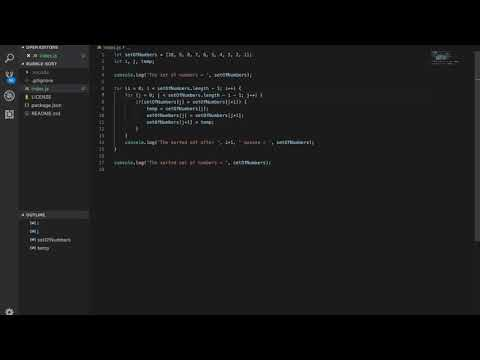View on GitHub

# Introduction

Bubble Sort is an algorithm that allows a list of elements to be put into order. This is done by swapping the elements that are out of order. Given a size of n elements, the algorithm runs O(n)^2 time.

## Pseudo Code

``````    Start at 1 for an index, called i.
Given a list of elements, called e. While i is less than the length of e, do the following for each element in the list.
For an index, j, start at 1. While j is less than the length of e - i, do the following;
If the element at e[j] is less than e[j + 1], then swap the values.
``````

### Video## Code

Check out the code on GitHub. Hopefully I can update to include the snippet in the page here soon.